## Data Structures and Algorithms 2, Fall 1998 Problem Set 4 (30 Nov - 2 Dec)

1. Determine the payoff value of the following game tree for the first player, using the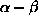pruning heuristic (p. 79 of the lecture notes).

(Figure omitted because of lack of space.)

2. Simulate the behaviour of the Lin-Kernighan 2-opt heuristic (p. 83 of the lecture notes) on the TSP instance below, starting with the initial candidate route abcde.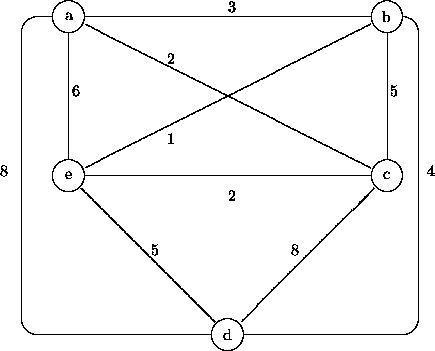3. Determine an optimal TSP route for the graph of Problem 2 using the branch-and-bound method presented on p. 81 of the lecture notes.
4. The NP-complete (i.e., difficult) PARTITION problem is defined as follows: given a set of 2n nonnegative integers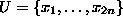, is there a subset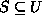of size n, such that the sum of the numbers in S is exactly half the sum of all the numbers in U? The problem can be reformulated as a minimization problem by taking as the cost of a candidate solution S to be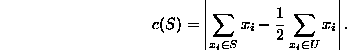The original decision problem now has answer ``yes'' if and only if there is a subsetsuch that c(S) = 0.

Implement, on your favorite computer system, one of the following three methods to solve this problem for a given fixed value of n: (a) iterated local search (from randomly chosen initial solutions), (b) simulated annealing, (c) some variety of genetic algorithm. Experiment with different parameter settings.

Pekka Orponen
Wed Nov 25 22:31:09 EET 1998